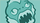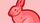cancel
Showing results for
Did you mean:

## Validate Credit card number

Hi, I am using the C# integration to do the payment, i want to know the minimum lenght of all credit card types to validate in my BLL method.Member
1 ACCEPTED SOLUTION

Accepted Solutions

Typically, credit card numbers are all numeric and the length of the credit card number is between 12 digits to 19 digits.

• 14, 15, 16 digits – Diners Club
• 15 digits – American Express
• 13, 16 digits – Visa
• 16 digits - MasterCard

For C#, the following validates a credit card number using the Luhn (Mod 10 algorithm):

```public static bool Mod10Check(string creditCardNumber)
{
if (string.IsNullOrEmpty(creditCardNumber))
{
return false;
}

int sumOfDigits = creditCardNumber.Where((e) => e >= '0' && e <= '9')
.Reverse()
.Select((e, i) => ((int)e - 48) * (i % 2 == 0 ? 1 : 2))
.Sum((e) => e / 10 + e % 10);

return sumOfDigits % 10 == 0;
}```

Certified Authorize.net developersTrusted Contributor
2 REPLIES 2

There have been cards issued that are only 12 characters. Most cards are 16 digits. The standard way of validating is to use the Luhn algorithm. There's a lot of free sample code out there with it that you can incorporate.Typically, credit card numbers are all numeric and the length of the credit card number is between 12 digits to 19 digits.

• 14, 15, 16 digits – Diners Club
• 15 digits – American Express
• 13, 16 digits – Visa
• 16 digits - MasterCard

For C#, the following validates a credit card number using the Luhn (Mod 10 algorithm):

```public static bool Mod10Check(string creditCardNumber)
{
if (string.IsNullOrEmpty(creditCardNumber))
{
return false;
}

int sumOfDigits = creditCardNumber.Where((e) => e >= '0' && e <= '9')
.Reverse()
.Select((e, i) => ((int)e - 48) * (i % 2 == 0 ? 1 : 2))
.Sum((e) => e / 10 + e % 10);

return sumOfDigits % 10 == 0;
}```